# The Politics of Climate Change (Anthony Giddens)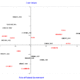###### US Presidential Inaugural Addresses (from 1957 to 2009)
20 February 2018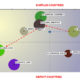###### The European Crisis according to Yanis Varoufakis
4 April 2018
T-LAB Tools for Text Analysis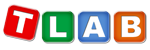www.tlab.it Short Samples: The Politics of Climate Change (Anthony Giddens) (February 20th, 2014. The version of T-LAB used was 9.1)

This short example consists of a sort of exercise through which, while analysing a popular book of the sociologist Anthony Giddens (i.e. The Politics of Climate Change), we focus on methodological issues concerning the use of some T-LAB tools for text analysis.

The book in question consists of an introduction, nine chapters and an afterword (see Fig. 1 below).
That means that - at the source - there are eleven ordered sections that the whole discourse of the author is subdivided into. Consequently, in T-LAB jargon, the book is a corpus which - via a categorical variable - is partitioned into eleven subsets.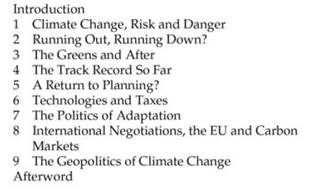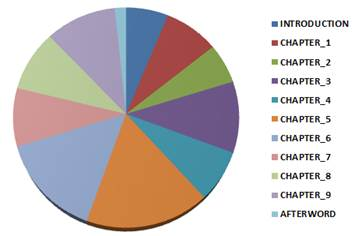Fig. 1

As a starting point (see section 1 below), we will explore some similarities and differences between these eleven subsets and we will map their relationships accordingly.

Subsequently (see section 2 below), by assuming that the various subsets (i.e. book chapters) exhibit the mains ‘themes’ (or ‘topics’) in different proportion, we will consider as analysis units text segments which roughly correspond to a couple of sentences (Click here for more information) and we will partition the book contents into thematic clusters consisting of such analysis units.

Finally (see section 3 below), by using some new features of T-LAB 9.1, the dynamic sequence of themes both within the entire book and their chapters will be explored.

SECTION 1: DEALING WITH BOOK CHAPTERS

A key point to keep in our mind is that – at every step of our exercise - each analysis unit (i.e. a book chapter, a text segment, a theme, etc.) can be represented as a feature vector, that is as a vector of term weights. And this is the very reason why lots of techniques for automated text analysis can apply algorithms for pattern recognition.

Actually, after the preprocessing phase, a contingency table is easily obtained (see Fig. 2 below), the rows of which correspond to key-words (i.e. terms) and the columns of which correspond to the sections that the Giddens book is subdivided into (i.e. eleven). So, in this case, each column is a vector the features of which (i.e. words) have a weight which corresponds to their occurrences within a chapter of the book. (N.B.: Depending on the type of analysis, various kinds of normalized weights can be obtained by using the T-LAB tools. For example, a clustering tool uses the TF-IDF and the Euclidean norm, the Correspondence Analysis tool uses the Chi-square distance, etc.).

More specifically, in our case, we use a list which includes 1,457 key words obtained through an automatic lemmatization process (e.g. the lemma ‘change’ includes all occurrences of distinct words like ‘change’, ‘changes’, ‘changing’, ‘changed’). As a ‘golden standard’, such a list doesn’t include stop-words (e.g. articles, prepositions etc.), but it does include word phrases and multi-word expressions like ‘global_warming’, ‘European_Commission’, ‘level_of_emissions’ and so on.
In our case, the lower occurrence value of the listed key-words is 5.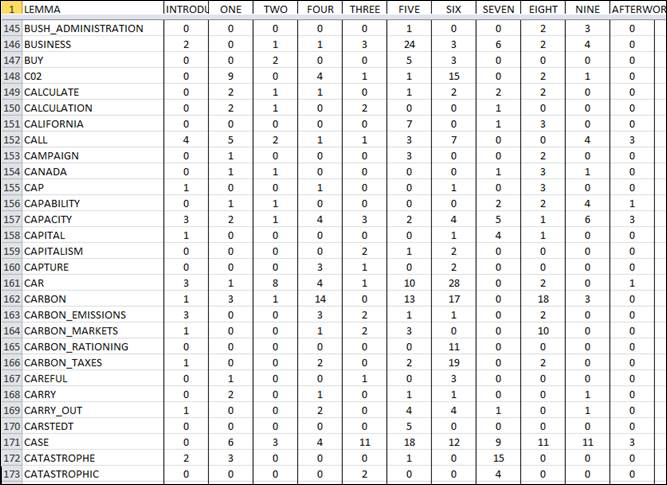In order to get a initial picture of the book contents, a simple Correspondence Analysis is performed which allows us to map the relationships between all rows and all columns of the above table, as well as to explore the hidden variables (i.e. the factors) which frame the Giddens discourse and - at the same time - refer to a sort of socio-cultural dialectics.

For example, the following two maps illustrate how both the relationships between corpus subsets and the relationships between key-words are rearranged through the semantic oppositions of the first two factors.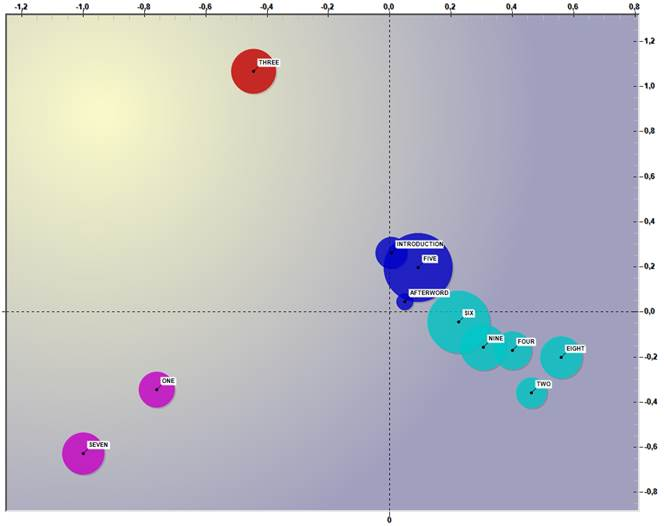Fig. 3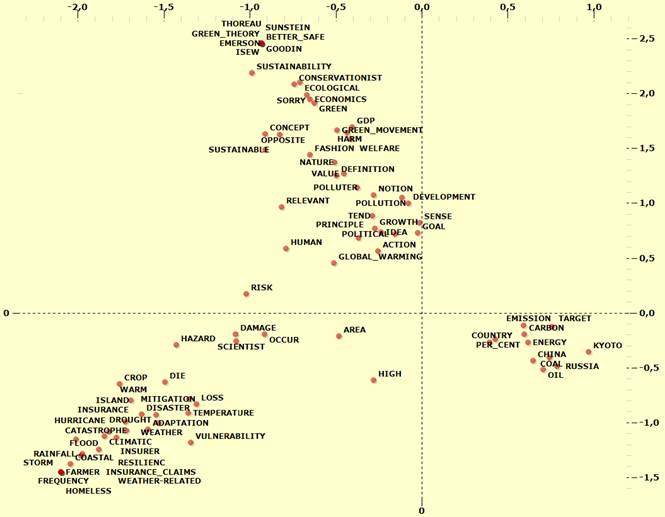Fig. 4

The semantic characteristics of the first two factors, respectively ‘X’ (abscissa, horizontal) and ‘Y’ (ordinate, vertical), and their oppositions are summarized by tables listing the absolute contributions of the characteristic words onto the factorial poles (see below).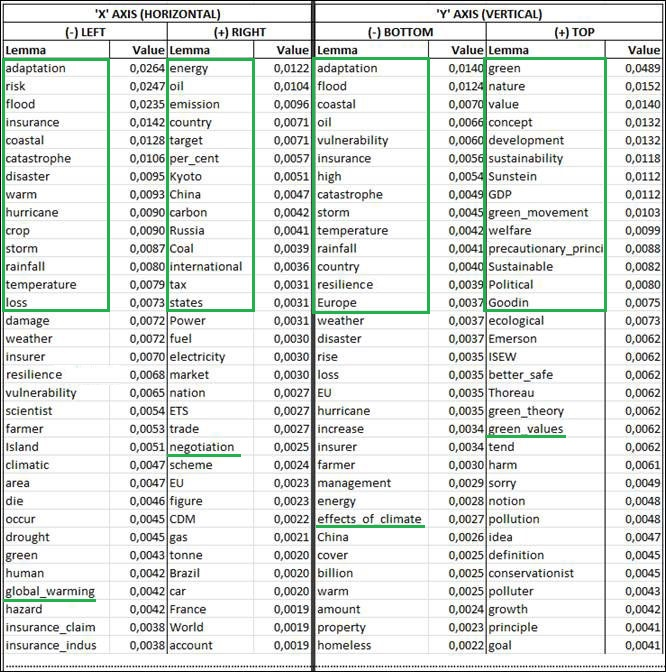Fig. 5

In short, we may say that the first bipolarity (i.e. the ‘X’ axis) concerns the ‘risks’ of the climate change on the left side and its ‘policy’ on the right side, whereas the second bipolarity (i.e. the ‘Y’ axis) concerns the experienced ‘effects’ on the bottom side and the values of ‘sustainable development’ on the top side.

Actually, because the shape produced by the first two factors resembles to a 'Y' sloping on the left side, there is a slight difference between 'risks' and 'effects'. So, as the ‘specific’ positions of some chapters on the map in Fig. 3 above are quite intriguing (see chapter three in the top-left quadrant, chapters one and seven in the bottom-right), we are interested in checking their characteristics. More specifically, by using the Specificity Analysis tool which applies the chi-square test to the intersections of the contingency table depicted in Fig. 2, we are enabled to list the ‘typical words’ of the above mentioned chapters.

Ordered by decreasing chi-square values, the top ten typical words of the three chapters in question (i.e. the words which, through a comparison with the entire corpus, result to be significantly ‘over used’ within these subsets) result to be the following:

 CHAPTER ONE CHAPTER THREE CHAPTER SEVEN IPCC (*) scientist warm sceptic temperature earth ice sea risk weather green nature concept value sustainability GDP development Sustainable precautionary_principle green_movement adaptation flood insurance coastal hurricane catastrophe crop loss insurer disaster

(*) ‘IPCC’ is the acronym of ‘Intergovernmental Panel on Climate Change’.

By computing normalized TF-IDF values, the above mentioned T-LAB tool allows us also to extract the most significant text segments of three chapters in question. In this case, just as an example, we report the first of each (i.e. those with the highest TF-IDF score).

CHAPTER ONE

Higher temperatures produce more acidity in the water, which could seriously threaten marine life. Warmer seas release more C02, accelerating the global_warming effect. As measured over the period from 1982 to 2006, temperatures rose most in the Baltic Sea (1. 35 °C), the North Sea (1. 3 °C) and the South China Sea (1. 22 °C).

CHAPTER THREE

In both senses, 'development' means the accumulation of wealth, normally measured in_terms_of GDP, such_that a society becomes progressively richer. It implies that this wealth is generated in some large part by the economic transformation of the society in question, as a self-perpetuating process.

CHAPTER SEVEN

During the winter of 2001-2 it was closed a record 24 times, as_a_result of historic highs in the freshwater levels of the river. Of the total stock of domestic dwellings in the UK, 10 per_cent is currently at risk of flooding. In the summer of 2007, the UK experienced the most intense rainfall known since records began, giving rise to widespread floods.

Before going further, it is worth recalling that some T-LAB tools allow us to explore the word co-occurrence relationships within each corpus subset.

For example, by selecting a short list of key words (i.e. those with an occurrence value => 10), the internal relationships within chapter three, can be plotted either by means of a MDS method (see Fig. 6 below) or by means of Network Analysis (see Fig. 7 below).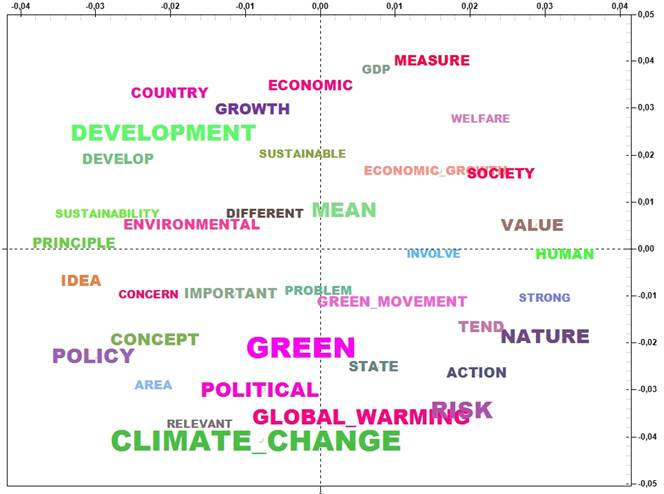Fig. 6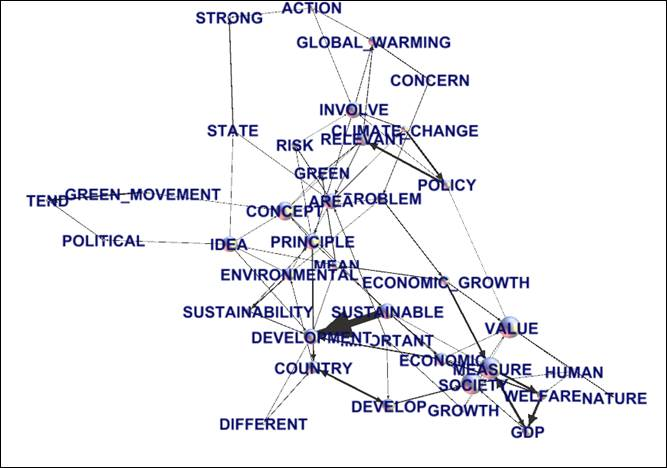Fig. 7

N.B.: The above graph has been realized through Gephi (http://gephi.org/) by importing a .gml file created by T-LAB.

SECTION 2: DEALING WITH THEMES

Let’s now try to perform a thematic analysis of the Giddens book.

Actually T-LAB includes a specific tool (i.e. Thematic Analysis of Elementary Contexts) which allows us to do this in a easy and straightforward way; however, by considering the didactic nature of this example, we have decided to proceed otherwise and provide the reader with all technical details.

As is known, when talking of ‘automated’ thematic analysis, if our aim is to assign each analysis unit to a fixed category (i.e. a theme), three key points must be clarified in advance:

a) which analysis units to consider;

b) which categories to use;

c) which algorithms to apply.

In relation to the above point (a) we use the text segments automatically provided by T-LAB (see, for example, the three characteristic elementary contexts quoted at the end of the previous section 1).

With regards to points ‘b’ and ‘c’, as both call into question the difference between supervised and unsupervised methods (i.e. between methods which use pre-defined categories and methods which seek patterns in the data), we use a hybrid approach consisting of the following steps:

1- two unsupervised methods are applied which allow us to extract the main themes of the book and to describe each of these themes by means of feature vectors. The two methods, both implemented in T-LAB, are: the bi-secting K-means clustering and the topic model.

More specifically, two different T-LAB tools ( i.e. the Thematic Analysis of Elementary Contexts , which uses the bi-secting K-means algorithm, and Modeling of Emerging Themes ,which uses the Latent Dirichlet Allocation and the Gibbs Sampling for the topic analysis) allow the user to export dictionaries the feature vectors of which consist of term weights obtained by using either the Chi-square values (unsupervised clustering) or the probability values (topic model).

2- two supervised classifications of text segments are performed: the first one by using the feature vectors describing the clusters, the second one by using the feature vectors describing the topics. More details will be provided below.

3- the results of the above two classifications are compared and one of them is used for further analyses.

In order to compare the results provided by the two unsupervised methods (see ‘1’ above), we decide to obtain the same number (i.e. twelve) of ‘themes’/’topics’, and so the same number of feature vectors describing each of them.

As explained in the T-LAB Manual/Help, in the case of supervised classification (see ‘2’ above), the analysis steps are the following:

a) normalization of the seed vectors corresponding to the 'k' categories of the dictionary used;

b) computation of Cosine similarity and of Euclidean distance between each 'i' context unit (i.e. text segment) and each 'k' seed vector;

c) assignment of each 'i' context unit to the 'k' class or category for which the corresponding seed is the closest (In this case, maximum Cosine similarity and minimum Euclidean distance must coincide, otherwise T-LAB consider the 'i' context unit as unclassified).

In our case the above three steps are repeated twice: the first time by applying the category dictionary obtained by the unsupervised clustering, the second time by applying the category dictionary obtained by the topic model.

Some measures provided by T-LAB (i.e. Calinski-Harabasz, Davies-Bouldin, McClain-Rao and Silhouette indexes) allow us to compare the two partitions obtained by the above mentioned unsupervised methods and – as a result - their quality doesn’t appear to be significantly different.
However, after having evaluated the semantic coherence of the two solutions, we decide to use the partition obtained by using the dictionary extracted through the bi-secting K-Means algorithm (i.e. the partition which is able to classify 83.73% of 1,604 text segments that the Giddens book has been subdivided into).

It is worth noting that, while both the ‘contents’ of the two partitions (i.e. the characteristic words of the various classes) and their distribution within the semantic space vary, the way they are framed into such a space is substantially the same
The T-LAB tool which allows us to assess such a result (i.e. similarity in framing) is the Correspondence Analysis performed after each of two above classifications has been obtained, i.e. by mapping two contingency tables, the rows of which have the same key-words as headers, whereas the column headers are different (i.e. ‘thematic clusters’ in the first case and ‘topics’ in the second one).

More specifically, the ‘meanings’ of the first two factors obtained in two cases appears to be substantially the same (Click here to see the absolute contributions obtained by analysing the contingency table including ‘themes’. Click here to see the absolute contributions obtained by analysing the contingency table including ‘themes’. N.B.: The fact that in the two cases the left/right and top/bottom polarities result to be inverted is just a geometric effect).

Having said that, let us summarize the characteristics of the chosen partition into thematic clusters.

Ordered according to their Chi-square values, the most relevant words of twelve themes are listed below (N.B.: Each theme is labelled by using some of its typical key-words. More specifically, even if T-LAB automatically suggests 'its' labels, in this case each one of them has been assigned manually by using a specific feature of the software).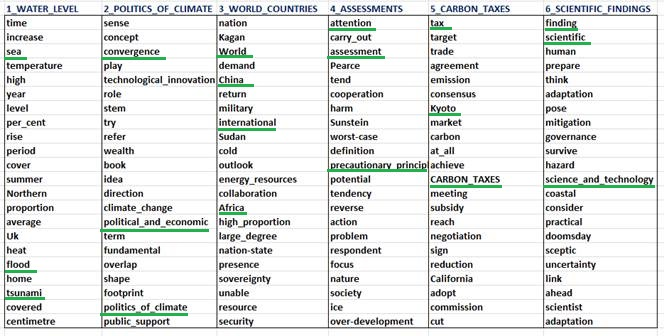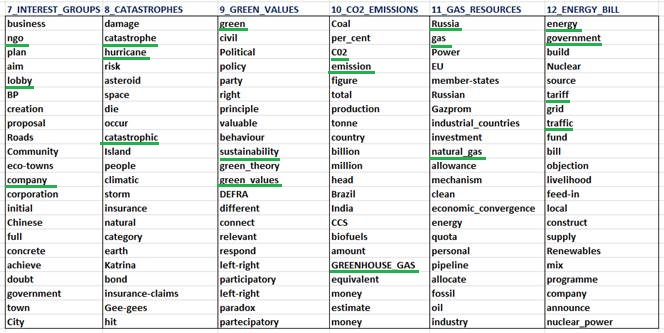Fig. 8

Click here to see the two most typical text segments of each thematic cluster (measure = chi-square test),
(N.B.: the listed text segments can also be used for a sort of text summarization).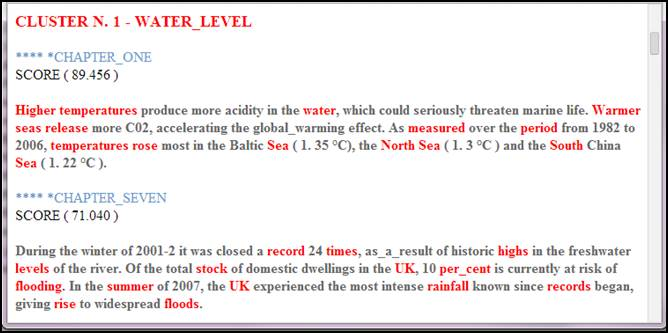The relative weights of the twelve thematic clusters, which correspond to the percentage of text segments falling into each of them, are summarized by the following chart.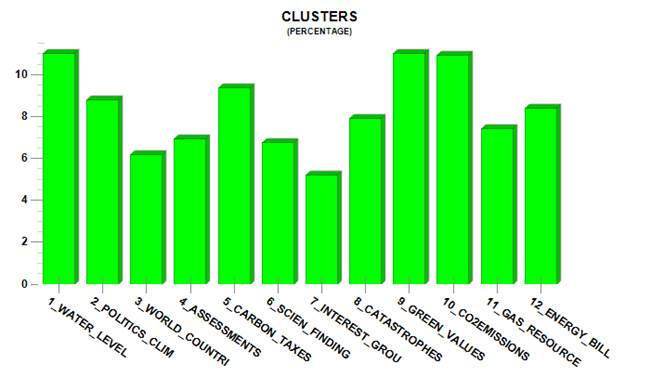Fig. 9

The way the twelve themes are framed into the semantic space of the first two factors is the following: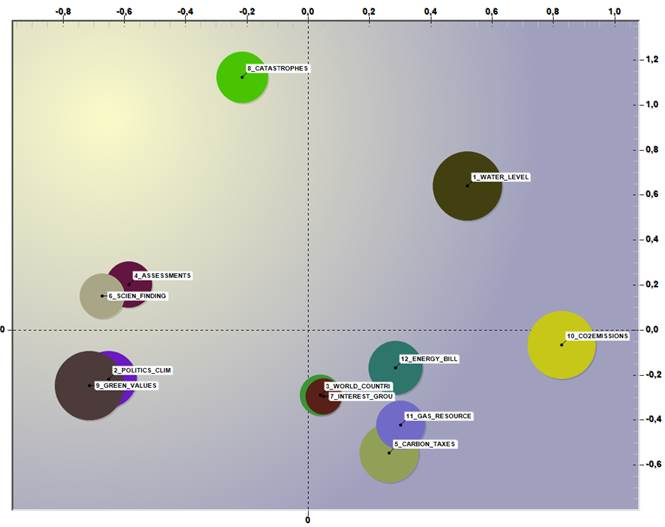Fig. 10

The twelve themes cross the eleven sections of the book is the following way: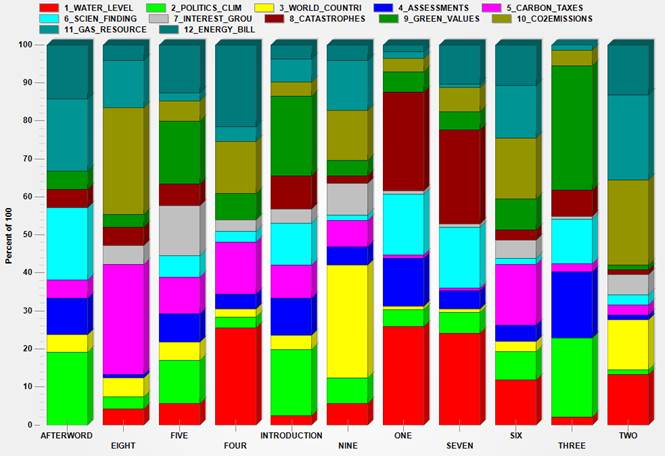Fig. 11

So, for example, the main themes of chapter eight (the title of which is 'International Negotiations, the EU and Carbon Market’) result to be ‘CO2_EMISSIONS’ and ‘CARBON_TAXES’.

SECTION 3: DEALING WITH THE SEQUENCES OF THEMES

Starting from the version 9.1, T-LAB includes a new tool which – when the corpus consists of subsets ordered in a sequential fashion (e.g. chapters of a book, parts of an interview, turns in a conversation or a debate, etc.) – allow us to map the sequences of themes in quite an interesting way.

To start with, let’s examine the following matrices (see Fig. 12 and Fig. 13 below) which cross the twelve themes with each other.

More specifically, the numbers in Fig. 12 indicate how many times each theme in a row precedes each theme in a column. For example, POLITICS_CLIMATE results to be a predecessor of GREEN_VALUES twenty-six times. That means that – according to the T-LAB analysis – 26 text segments classified as belonging to the GREEN_VALUES theme are successors of text segments classified as belonging to the POLITICS_CLIMATE theme.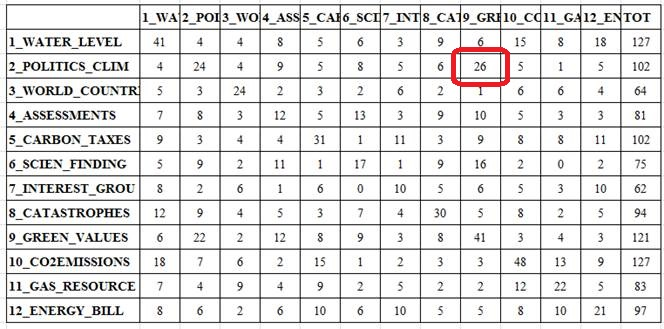Fig. 12

As one could intuit, the most frequent cases are those where both the predecessor and the successor refer to the same theme (see the diagonal of both matrices). In fact, when ‘engaged’ in a specific theme, arguably the author has spent more than a couple sentences (and one after the other) on such a theme.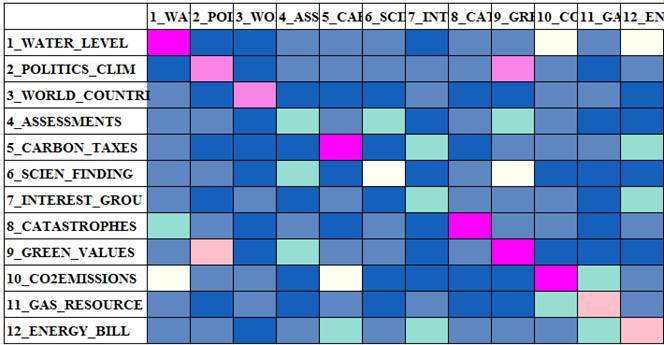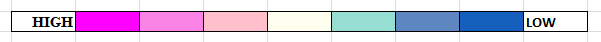Fig. 13

In other words, such a tool allows the user to perform a specific kind of ‘discourse analysis’ which takes into account the theme sequences.

Actually these kinds of sequences can also be tracked by means of animated charts either referring to the entire corpus or to a subset of it.
For example, by clicking the below pictures it is possible to track how the thematic discourse evolves within chapter three of the Giddens book.

More specifically:

- the 3d matrix, which crosses the twelve themes with each other, shows how each transition (i.e. predecessor --> successor) increases over time;

- the 2d chart, the abscissa and the ordinate of which correspond to the factorial axes selected by the user, shows how the dimension (i.e. percentage) of each theme varies over time. Meanwhile moving arrows indicate how themes follow each other.

Fig. 14

Fig. 15

Last but not least, as T-LAB allows the user to save some files (e.g.: .dl, .gml, .net, .vna formats) which can be easily imported by software for network analysis like Gephi (http://gephi.org/) and many others, a graph like the following can be quickly obtained.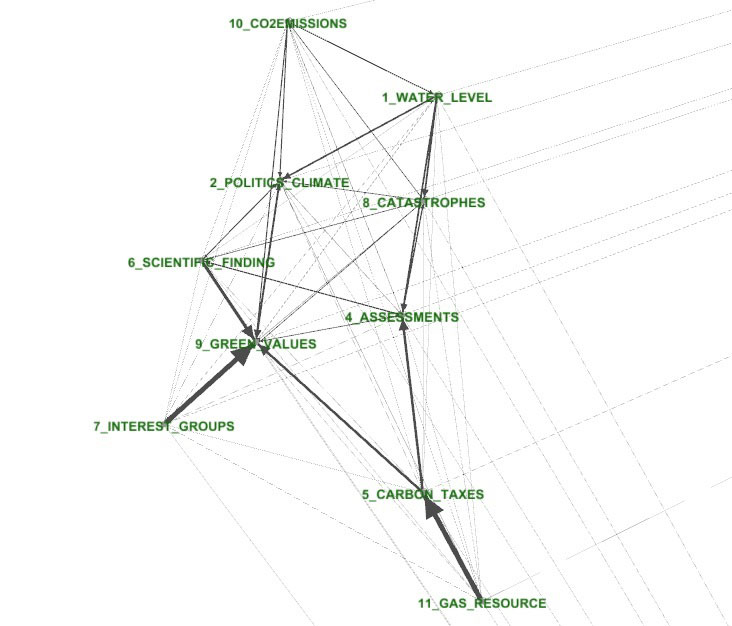Fig. 16

REFERENCES

Giddens, Anthony (2009). The Politics of Climate Change. Cambridge: Polity Press
Lancia, Franco (2012). The Logic of T-LAB Tools Explained. Retrieved from https://www.tlab.it/en/toolsexplained.php